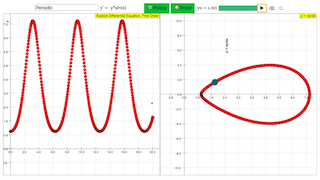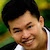Description

First order differential equation

The simulation calculates solutions of ordinary explicit first order differential equations

y´ = dy/dx = f(y,x)

using the Runge−Kutta algorithm. In the left coordinate system the abscissa represents x, the ordinate y.

When opening the file you see a fat red point at x = 0, representing the initial value y0 at its abscissa x0 . You can change the initial value with the slider, more exactly and unlimited by typing a value into the number field. Two additional number fields are used to define x0 and xmax . Default values are: y0 = 1; x0 = 0; xmax = 3. You can also draw the red point to create new initial conditions.

In the comboBox you can chose between a number of predefined types of functions. Their formula is shown in field y´ = .. . There you can edit formulas or input any arbitrary first order explicit differential equation.

When activating start with the default equation, the differential equation of the exponential function y´= y is evaluated. Calculation stops as x = xmax . At first you see a set of calculated points. You can choose the option trace to get an interpolated smooth curve.

Stop stops the calculation; back leaves already calculated points and sets back to the initial conditions. Changing these now creates an additional curve at start. This way you can generate sets of solutions for different initial conditions (the Trace option would create jumps, which are avoided in the points option). Clear resets and clears traces, but leaves parameters unchanged. Reset leads back to default values.

After back you can change the x-resolution of the calculation by slider step, and look if and how different resolution influence the result.

The smaller window shows the phase space projection

y ´ = y´ ( y )

The green point is the one last calculated.

The phase space diagram very distinctively demonstrates the different character of solutions:  convergence, divergence, periodic oscillation, oscillating divergence, oscillating convergence. It is independent of the initial condition. The order of predefined functions follows these characteristics.

Calculus

Numerical integration of differential equations with EJS

Using EJS it is very easy to solve differential equations. Several algorithms for different methods are programmed and can be chosen at page Evolution with a mouse click. The steps of the variable x are automatically calculated, when the difference dx has been defined. For a first order differential equation one defines:

dy/dt = y´ = f( y, x )

In this simulation with a ComboBox the formula is:

= (formula in field , evaluated for x, and y)

In February 2011 EJS 4.3 presents the following methods:

• Euler
• Euler−Richardson
• Velocity Verlet
• Runge− Kutta, 4- steps
• Bogacki−Shampine 3(2)
• Cah−Karp 5(4)
• Fehlberg 8(7)
• Dormand−Prince 5(4)
• Dormand−Prince 8(5
• OSS3
Experiments

E 1:  Run exponential function and try the points and the trace option. After back try different step widths (speed). With the exponential you will see no difference within the given range of the slider.

E 2: Go back, and chose a new initial condition. Start creates the solution, which is different from the first one. Try the points and trace options.

E 3:  Create a set of solutions with identical x0 and different y0. Explain what you see.

E 4: Create a set of solutions with identical y0 and different x0. Why do you see curves that are shifted parallel?

E 5: Create sets of solutions including negative initial values y0. Discuss the result as consequence of the differential equation.

E 6:  Choose exponential damping. Compare solutions for positive and negative initial values to those of the exponential.

E 7:  Choose any of the oscillating functions and try to guess the trace before calculation by studying the differential equation.

E 8: Edit the exponential by adding factors g(x), especially periodic ones. Try to understand what you observe.

E 9: Run the exponential once. Choose back. Edit the formula by setting e^x instead of y. In a second run you will see the same result:  The algorithm now performs ordinary Integration (numerical calculation of the antiderivative = indefinite integral). This works for the exponential, because y is not explicit in dy/dx = e^x.

.

E 10: Choose any other function not containing y, e.g. sin(x). For this you get -cos(x) as indefinite integral,with the initial value as integration constant. Compare the phase space diagram of Sine with that of the function type Periodical.

E11:  Choose constant velocity and create a curve set for different factors y, including zero. This again is a simple integration. Edit the differential equation in such a way that an initial starting location (x0) is considered.

E12: Choose constant acceleration and create a curve set for different factors of y, including zero. This again is a simple integration. Edit the differential equation in such a way that an initial velocity is considered.

E 13:  Extend the differential equation by more complicated functions of y and let yourself be surprised by what happens. Try to define and understand interrelated families of differential equations. Are there more classes than convergent, divergent, periodic?

E14:  After back change the step width for different functions and observe the result. Which characteristics define the maximum width below which no deviations are observed?

Authors

This file was created by Dieter Roess in March 2009

This simulation is part

– Plus 2000 Examples from Physics”

ISBN 978-3-11-025005-3, Walter de Gruyter GmbH & Co. KG

Translations

Code Language Translator RunSoftware Requirements

SoftwareRequirements

 Android iOS Windows MacOS with best with Chrome Chrome Chrome Chrome support full-screen? Yes. Chrome/Opera No. Firefox/ Samsung Internet Not yet Yes Yes cannot work on some mobile browser that don't understand JavaScript such as..... cannot work on Internet Explorer 9 and below

CreditsDieter Roess - WEH- Foundation; Tan Wei Chiong; Loo Kang Wee

[text]

For Teachers

This simulation provides a visualisation of first-order ordinary differential equations, available from a selection in the combo box provided. A total of 9 different types of equations can be selected and graphed on the plotting panel in the simulation.

The display of the plot can be toggled between Points (discrete points) and Trace (a continuous curve) in the combo box to the right of the formula box.

The initial starting point of y can be toggled with the slider labelled y0.

There are 2 plotting panels. The one on the left represents the graph of y against x, while the one on the right represents the graph of the derivative y' against y.

The available functions along with their associated differential equations are as follows:

- Exponential (y = e^x): y' = y

- Exponential Damping (y = e^(-x)): y' = -y

- Periodic: y' = ysin(x)

- Oscillation Divergent: y' = y(x/5)sin(x)

- Oscillation Convergent: y' = (y/x)sin(x)

- Oscillation Quadratic: y' = ysin((x-10)^2)

- Sine Exponent: y' = y^sin(x)

- Constant Velocity: y' = 1

- Constant Acceleration: y' = x

Research

[text]

[text]

[text]